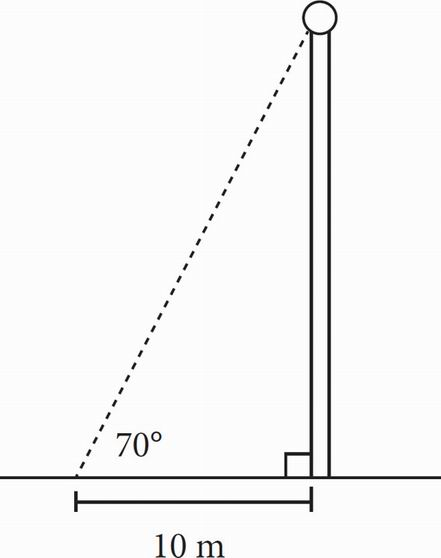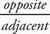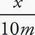# SAT Math Multiple Choice Question 57: Answer and Explanation

### Test Information

Question: 57

3.Martin wants to know how tall a certain flagpole is. Martin walks 10 meters from the flagpole, lies on the ground, and measures an angle of 70° from the ground to the base of the ball at the top of the flagpole. Approximately how tall is the flagpole from the ground to the base of the ball at the top of the flagpole?

• A. 3 m
• B. 9 m
• C. 27 m
• D. 29 m

C Use SOHCAHTOA and your calculator to find the height of the flagpole. From the 70° angle, you know the adjacent side of the triangle, and you want to find the opposite side, so you need to use tangent. Tangent, so tan 70° =, where x is the height of the flagpole up to the ball. Isolate x by multiplying both sides by 10: 10 tan 70° = x. Use your calculator to find that 10 tan 70° = 27.47, which is closest to (C).